# Energy of translation compared to the energy of rotation

I use an example with a rack and a pinion. I suppose there is no losses from friction. I suppose the masses very low to simplify the study, and there is no acceleration. I suppose the tooth of the pinion and the rack perfect, I mean there is no gap. There is always the contact between the rack and the pinion, I mean the tooth are always in contact.

The device: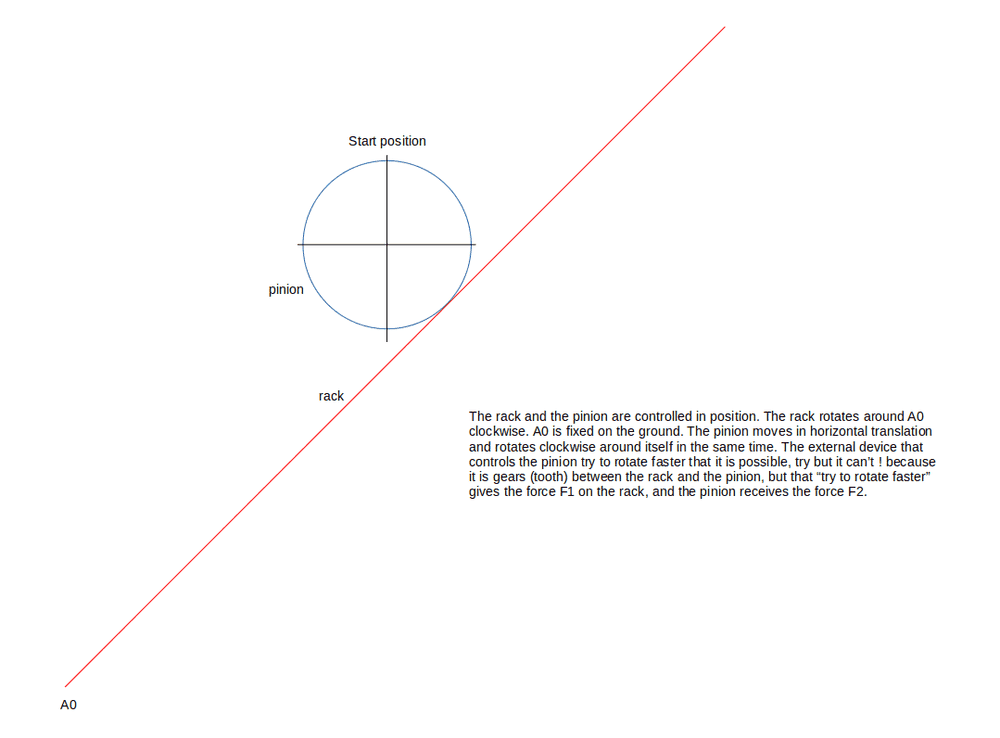I drew 2 positions: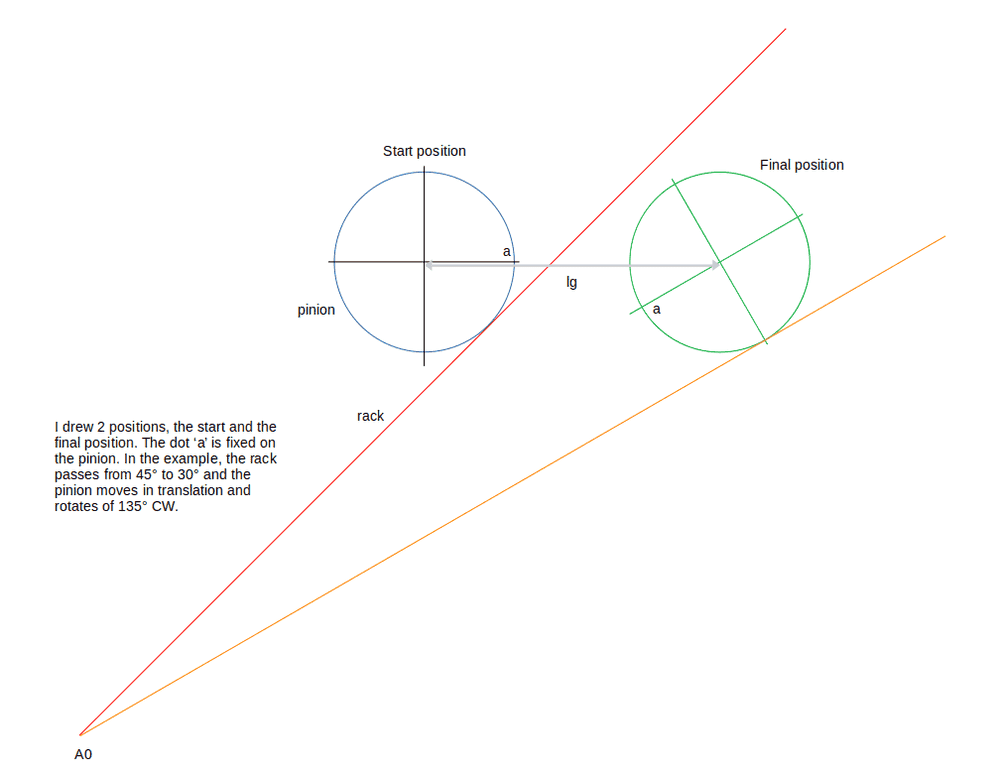The distance lg equal to 131 mm.

Because the pinion tries to rotate faster there are the force F1 on the rack and the force F2 on the pinion: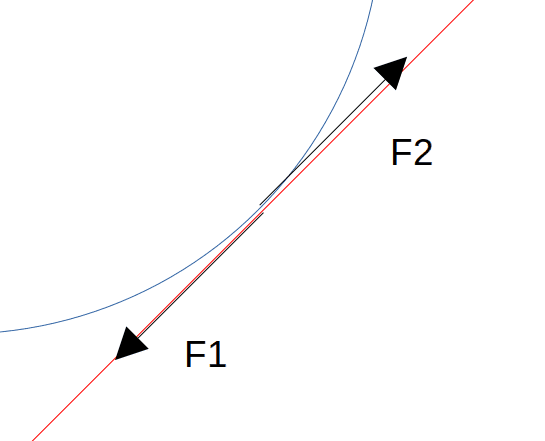I suppose the force F1 and F2 constant in value for the calculation, the value of F1 = the value of F2 = 1 N for example. The value of F1 is equal to the value of F2. The orientation of the forces F1 and F2 have the orientation of the red wall.

I used a geometric method to measure the distance of rotation needed for the pinion (around itself):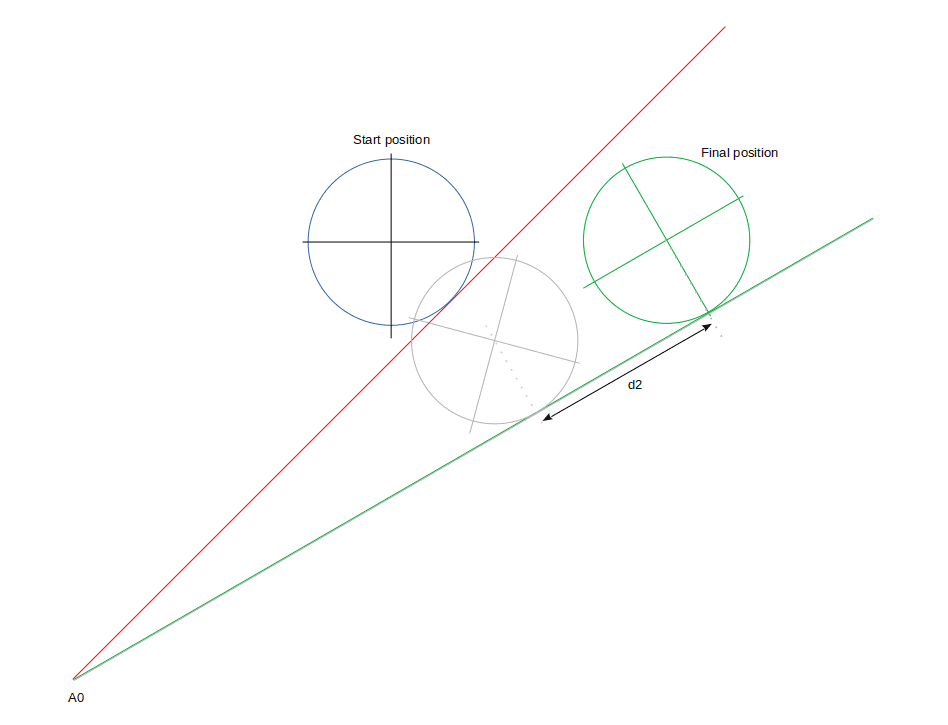I measured d2=94 mm. So the energy needed to rotate the pinion is d2*F1 = 94*1= 94 J. The energy needed to move the pinion in translation is lg*(sin(pi/4)-sin(pi/6))/(pi/12) = 103 J.

So maybe the distance d2 is not like I think ? or the integral is not correct ?

I used a program to measured the length d2 and lg and the energies:

Code:
#include <stdio.h>
#include <math.h>

int main()
{
long double pi=M_PI;
long int N=10000000L;
long double R=.1;
long double D=1.;
long double xinf=1;
long double xsup=xinf/tanl(44.99/180.0*pi);

long double px1=0,py1=0,a1=0,px2=0,py2=0,a2=0,dl=0,wrotation=0,wtranslation=0,ix1=0;
long double iy1=0,ix2=0,iy2=0,qx1=0,qy1=0,s=0,xl1=0,yl1=0,xl2=0,yl2=0;
long double sx=0,sy=0,qx2=0,qy2=0,suma=0,l=0,asav=0;
long double xf=0,yf=0,wp=0,af=0,dlrc=0,dlpc=0;
long int i;
qy1=D;
py1=qy1-R;

for(i=0;i<N;i++)
{
qx1=xinf+(xsup-xinf)/N*i;
a1=atanl(qy1/qx1); if(i==0) a2=a1;
s=R/tanl(a1/2.);
px1=qx1-s;
ix1=px1+R*sinl(a1);
iy1=py1-R*cosl(a1);

if(i>0)
{
l=(sqrtl(ix1*ix1+iy1*iy1)+sqrtl(ix2*ix2+iy2*iy2))/2.;
xf=ix2+l*sinl(a1)*fabsl(a2-a1);
yf=iy2-l*cosl(a1)*fabsl(a2-a1);
af=fabsl(atanl((iy1-yf)/(ix1-xf)));
wtranslation+=fabsl(px2-px1)*cosl((a1+a2)/2);
wrotation+=sqrtl((ix1-xf)*(ix1-xf)+(iy1-yf)*(iy1-yf))*cos(a1-af);

}
px2=px1;  py2=py1;  qx2=qx1;  qy2=qy1;  ix2=ix1;  iy2=iy1;  xl2=xl1;  yl2=yl1;  a2=a1;

}
printf("\nN=%ld , R=%Lf , D=%Lf , xinf=%Lf , xsup=%Lf",N,R,D,xinf,xsup);
printf("\nwtranslation=%Lf , wrotation=%Lf\n",wtranslation,wrotation);
return 0;
}

And the notations: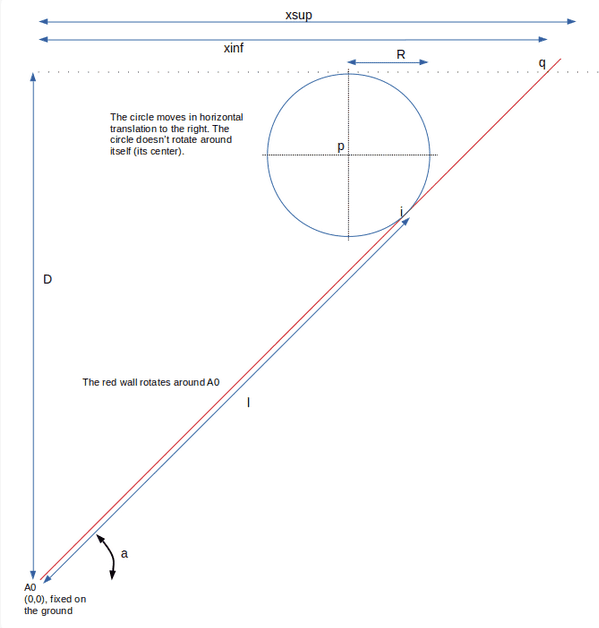kuruman
Homework Helper
Gold Member
Are you trying to determine how the rack should be rotated so that the pinion is at fixed height?

Are you trying to determine how the pinion should be rotated so that the wheel is at fixed height?
Yes, it is a part of the question. I'm not sure about the rotation of the pinion. But I'm not sure also in the calculation of the energies in translation and in rotation.

A.T.
I used a geometric method...
How about trigonometry and properly formatted formulas?

•JrK
How about trigonometry and properly formatted formulas?
I like you AT, you're like a good professor :)

I noted ##i## the dot of intersection between the pinion and the rack, ##p## the dot of the center of the circle. I added ##x## and ##y## for easting/norhting and I added ##1## and ##2## to represent the last position ##2## and the new position ##1##. The radius of the pinion is ##R##. The height from ##A_0## to the top of the pinion is ##D##. The angle of the rack relatively to the horizontal is ##\alpha##.

##i_{y2} = D-R-Rcos(\alpha_2)##
##i_{x2} = i_{y2}/tan(\alpha_2)##
##p_{x2} = i_{x2}-Rsin(\alpha_2)##

##i_{y1} = D-R-Rcos(\alpha_1)##
##i_{x1} = i_{y1}/tan(\alpha_1)##
##p_{x1} = i_{x1}-Rsin(\alpha_1)##

##d_2 = \sqrt{i_{x2}^2+i_{y2}^2} - \sqrt{i_{x1}^2+i_{y1}^2} ##
##d_1 = p_{x1} - p_{x2} ##

The energy from the translation is ##d_1 \int_{\frac{\pi}{4}}^{\frac{\pi}{6}} F cos(\alpha) d\alpha##

Numerical application : I take ##F_1=F_2=1 N##

##\alpha_2=45°##
##i_{y2} = 181.71##
##i_{x2} = 181.71##
##p_{x2} = 153.43##

##\alpha_1=30°##
##i_{y1} = 175.36##
##i_{x1} = 303.73##
##p_{x1} = 283.73##

##d_2 = 93.74##
##d_1 = 130.3##

The work from the rotation is ##F*d_2 = d_2 = 93.74##

The energy from the translation is ##d_1 \frac{\sin(\frac{\pi}{4}) - \sin(\frac{\pi}{6})}{\frac{\pi}{12}} = 0.791*130.3 = 103.08##

Maybe the energy from translation is more ##\int(10*(4*cos(x)-21)*csc(x)^2)*cos(x) dx## from ##\frac{pi}{4}## to ##\frac{pi}{6}## it is 104.2 J it is closer than the value I have with the program.

In the numerical application, the values used in the program is not the same than the values I used in the measured. I choose the value of the drawing: ##R=40## and ##D=250##, ##xinf=250## and ##xsup=433##. I verified each measure with the drawing.

It is better to have the same, so I corrected my program:

Code:
#include <stdio.h>
#include <math.h>

int main()
{
long double pi=M_PI;
long int N=10000000L;
long double R=40;
long double D=250;
long double xinf=250;
long double xsup=433;//xinf/tanl(30/180.0*pi);

long double px1=0,py1=0,a1=0,px2=0,py2=0,a2=0,dl=0,wrotation=0,wtranslation=0,ix1=0;
long double iy1=0,ix2=0,iy2=0,qx1=0,qy1=0,s=0,xl1=0,yl1=0,xl2=0,yl2=0;
long double sx=0,sy=0,qx2=0,qy2=0,suma=0,l=0,asav=0;
long double xf=0,yf=0,wp=0,af=0,dlrc=0,dlpc=0;
long int i;
qy1=D;
py1=qy1-R;

for(i=0;i<N;i++)
{
qx1=xinf+(xsup-xinf)/N*i;
a1=atanl(qy1/qx1); if(i==0) a2=a1;
s=R/tanl(a1/2.);
px1=qx1-s;
ix1=px1+R*sinl(a1);
iy1=py1-R*cosl(a1);

if(i>0)
{
l=(sqrtl(ix1*ix1+iy1*iy1)+sqrtl(ix2*ix2+iy2*iy2))/2.;
xf=ix2+l*sinl(a1)*fabsl(a2-a1);
yf=iy2-l*cosl(a1)*fabsl(a2-a1);
af=fabsl(atanl((iy1-yf)/(ix1-xf)));
wtranslation+=fabsl(px2-px1)*cosl((a1+a2)/2);
wrotation+=sqrtl((ix1-xf)*(ix1-xf)+(iy1-yf)*(iy1-yf))*cos(a1-af);

}
px2=px1;  py2=py1;  qx2=qx1;  qy2=qy1;  ix2=ix1;  iy2=iy1;  xl2=xl1;  yl2=yl1;  a2=a1;

}
printf("\nN=%ld , R=%Lf , D=%Lf , xinf=%Lf , xsup=%Lf",N,R,D,xinf,xsup);
printf("\nwtranslation=%Lf , wrotation=%Lf\n",wtranslation,wrotation);
return 0;
}

Last edited:
A.T.
The work from the rotation is

The energy from the translation is
Is "work from the rotation" supposed to be the energy input, and "energy from the translation" the energy output?

Is "work from the rotation" supposed to be the energy input, and "energy from the translation" the energy output?
Yes, but maybe I forgot one work.

A.T.
Yes, but maybe I forgot one work.
How did you derive the formula for translation work?

How did you derive the formula for translation work?
I think my formula is approximative but not to far from the real result I found with the numerical integration: I directly multiply the total distance moved by the center of the circle by the integrate of ##cos(\alpha)## and I found the mean with the sinus function. But I think it is not perfect and works only for a small angle of integration, so after I used Wolfram and I derivated the ##p_x## and I integrated the derivate multiply by ##cos(\alpha)##, and I found the exact value I found with the program. I updated the work in the previous message. I don't know how to have the work directly from ##p_x = \frac{D-R-Rcos(\alpha)}{\tan(\alpha)}-Rsin(\alpha)## when I integrate from start to end with the angle the value is bad.

A.T.
I think my formula is approximative but not to far from the real result I found with the numerical integration: I directly multiply the total distance moved by the center of the circle by the integrate of ##cos(\alpha)## and I found the mean with the sinus function. But I think it is not perfect and works only for a small angle of integration, so after I used Wolfram and I derivated the ##p_x## and I integrated the derivate multiply by ##cos(\alpha)##, and I found the exact value I found with the program. I updated the work in the previous message. I don't know how to have the work directly from ##p_x = \frac{D-R-Rcos(\alpha)}{\tan(\alpha)}-Rsin(\alpha)## when I integrate from start to end with the angle the value is bad.
Sounds messy.

Also, the rotation work is just angle * torque. No idea why you involve d2 there.

Sounds messy.
I need a little help there. From ##p_x = \frac{D-R-Rcos(\alpha)}{\tan(\alpha)}-Rsin(\alpha)## how to calculate the integration without pass from the derivative function ?

Also, the rotation work is just angle * torque. No idea why you involve d2 there.
I used the length by the force (like I could move in translation the rack). But, it is the same result with the torque by the angle ##RF*135/180*\pi = 40*2.35*1 = 94##

Last edited:
kuruman
Homework Helper
Gold Member
What I don't understand is why the angle labeled ##a## in your drawings would change. I assume that the pinion is powered by some energy source. It can only exert a force parallel to the rack with no perpendicular component and you show exactly that in your original post. What then would provide the necessary torque about the pivot to change the angular state of motion of the rack? Am I missing something?

What I don't understand is why the angle labeled a
##a## is a fixed dot on the pinion. Torque about the pivot, you mean A0 ?

AT: in fact, I didn't think with a torque on the pinion, because it moves in translation, so I thought there is no energy needed/recover from the rotation of the pinion. The angle of rotation of the pinion is well near 150° not near 135° like I thought because I looked for the geometric transformation, in that case the work from the rotation is equal to the work of the translation. But it was not like that I thought. I can think with the energy recovered from the translation of the pinion and with the translation of the rack no ? Ok, it is not exactly what I explained, but why if I move the rack along the wall (the line I drew), the energy seems to be d2*F ? I mean why I cannot push the pinion, push the rack along the red line and let the pinion rotate itself ? it is a blockage ?

In that case, the final position is more like that (green color):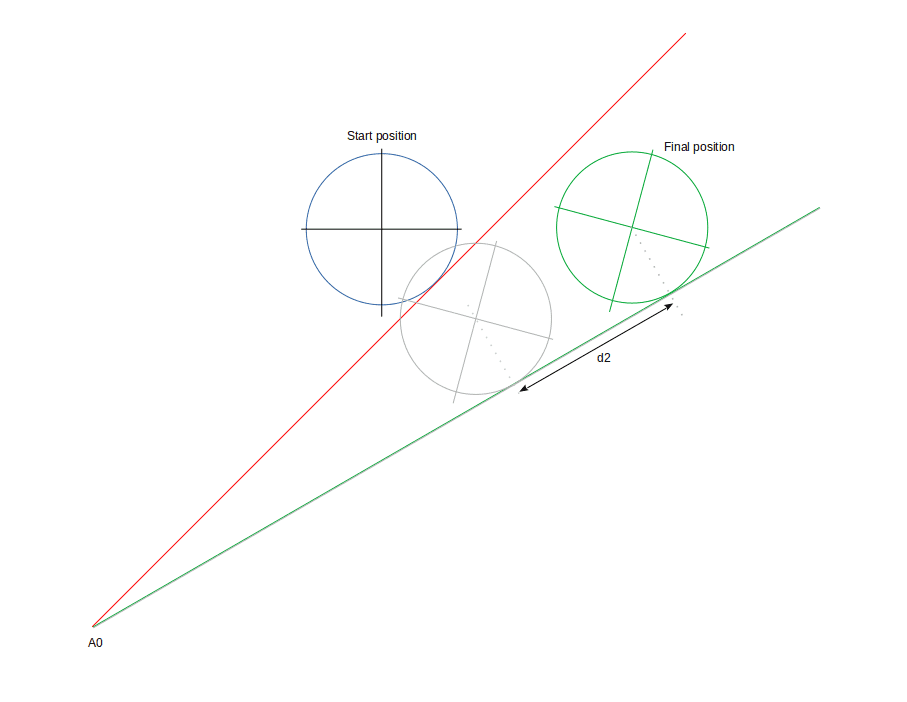Last edited:
kuruman
Homework Helper
Gold Member
##a## is a fixed dot on the pinion. Torque about the pivot, you mean A0 ?
Look at the last drawing in post #1. Next to label A0 you have defined an angle ##a## with a double arrow. Obviously you think that this angle is changing because you indicate that it is in post #13. In an effort to understand this contraption and perhaps help you, I am asking you: what force generates the torque about A0 that is necessary to make this angle change? Is gravity acting conventionally from the top of the screen to the bottom? If so, you do not mention it anywhere.

Look at the last drawing in post #1. Next to label A0 you have defined an angle with a double arrow.
Oh, yeah, sorry, the last drawing of the first message was for the notations of the program only but like in the program I can't use ##\alpha## in the program, so I used ##a##. I should have changed the name of the dot. No gravity here, and I think with masses very low. For me, ##a## is a dot fixed on the pinion to show the angle of rotation of the pinion.

AT: There are 2 manners to think. First, I translate the pinion and I rotate it, and the angle of rotation like I drew is well near of ##150°## so the energy recovered from the translation of the pinion is well the energy needed to rotate it: the program and the calculations are ok. Second method, I move the pinion in translation, I don't care about the rotation: I let him rotate like it wants and I move in translation the rack along the red line of the distance ##d_2##. The device that controls the pinion in translation applies a horizontal force (to the left) on the center of the pinion only. To move the rack along the red line I need an energy, and it seems that energy is lower. So maybe it is not possible to have that movement ?

The dot ##a## rotates like that in the second method :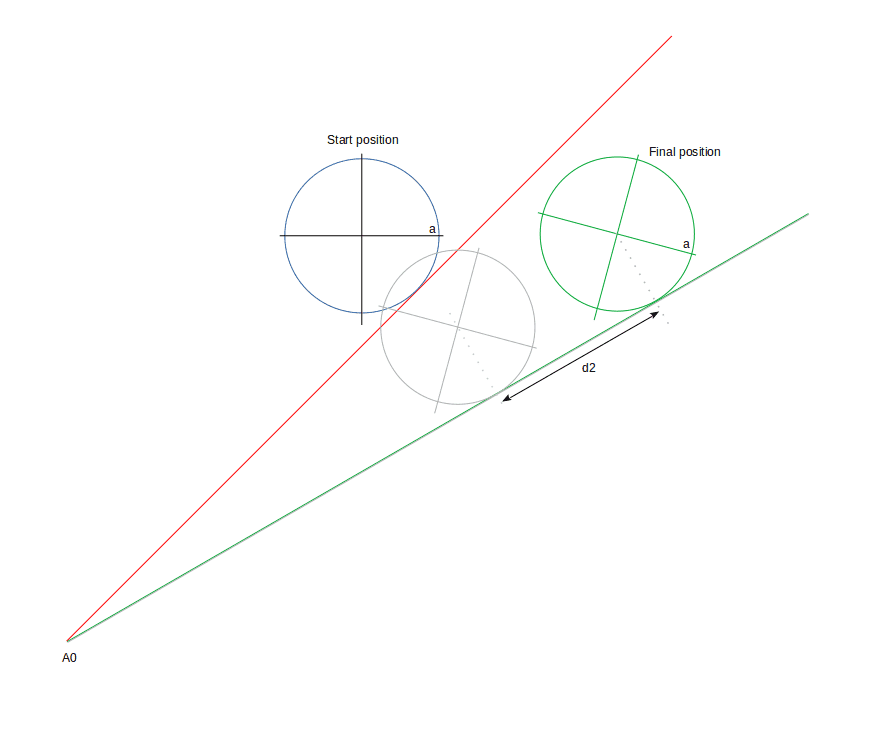Last edited:
kuruman
Homework Helper
Gold Member
Oh, yeah, sorry, the last drawing of the first message was for the notations of the program only but like in the program I can't use ##\alpha## in the program, so I used ##a##. I should have changed the name of the dot. No gravity here, and I think with masses very low. For me, ##a## is a dot fixed on the pinion to show the angle of rotation of the pinion.
I will ask you one last time. What causes the rack to rotate? You can calculate and simulate anything you want with your program but it will not correspond to a physical system unless it obeys the laws of physics.

What you have shown and explained so far indicates that the rack will not rotate about point A0; the final position of the pinion will not be as you show but farther away from A0 along a fixed rack. The green line and the red line will be on top of each other.

If the end of the rack at A0 is free to move, both the rack and the pinion will translate so as to keep their center of mass fixed in space. In this case the energy that goes in, presumably by a motor driving the pinion, will be split between the rack and the pinion.

If the end of the rack is not free to move, the rack will be fixed in space and the pinion will move away from the fixed end. In this case the energy goes entirely in the pinion.

A.T.
What then would provide the necessary torque about the pivot to change the angular state of motion of the rack?
If we assume that the rack is mass-less, you don't need a torque to rotate it.

A.T.
I need a little help there.
The simplest way is using the rest frame of the wall. Since everything is mass-less, you don't need to worry about inertial forces, which would normally occur in a rotating frame.

kuruman
Homework Helper
Gold Member
The simplest way is using the rest frame of the wall. Since everything is mass-less, you don't need to worry about inertial forces, which would normally occur in a rotating frame.
If everything is massless, what is the meaning of kinetic energy?

A.T.
If everything is massless, what is the meaning of kinetic energy?

--
No, I don't think about the kinetics energy, mass-less if possible or the mass as lower as possible. It is just to compare the energy.

The simplest way is using the rest frame of the wall.
Sure, in that case all is fine.

vanhees71
Gold Member
The simplest way is using the rest frame of the wall. Since everything is mass-less, you don't need to worry about inertial forces, which would normally occur in a rotating frame.
If it were massless you'd not have a rigid rod to begin with, or if you use the somewhat artificial model of a Born rigid body, it just rotates forever with constant angular velocity. You cannot change its rotation without making it in fact an elastic body. I've, however, no clue how you make a Born rigid body massless. My feeling is that this is so artificial a construction that it cannot formulated in any sensible way ;-)).

A.T.
If it were massless you'd not have a rigid rod to begin with, or if you use the somewhat artificial model of a Born rigid body, it just rotates forever with constant angular velocity. You cannot change its rotation without making it in fact an elastic body. I've, however, no clue how you make a Born rigid body massless. My feeling is that this is so artificial a construction that it cannot formulated in any sensible way ;-)).
This is the classical physics sub-forum. Masses rods are in heavy use here.

The point is simply that the rack & pinion neither store nor dissipate energy, so work in must equal work out.

jbriggs444
Homework Helper
If it were massless you'd not have a rigid rod to begin with, or if you use the somewhat artificial model of a Born rigid body, it just rotates forever with constant angular velocity. You cannot change its rotation without making it in fact an elastic body. I've, however, no clue how you make a Born rigid body massless. My feeling is that this is so artificial a construction that it cannot formulated in any sensible way ;-)).
As long as we have something somewhere to absorb the excess energy, I do not see how slowly moving, nearly rigid and negligibly massive objects are problematic.

However, from the original post, I do not see that anything has been specified to absorb the energy.

We are told that there are "no losses from friction". Also that there is "no acceleration". [Which is a bit problematic because if the wheel translates uniformly then the rack will accelerate and if the rack rotates uniformly then the pinion will accelerate. And if the rack rotates at all, it has bits which are accelerating]. It sounds like a clause designed to eliminate changes in kinetic energy. But since the apparatus is negligibly massive, kinetic energy is negligible anyway.

We are told that the pinion is "externally controlled". We are told that it translates horizontally ("moves in horizontal translation").

My interpretation would be that:

1. There is an input of torque to the pinion from the controlling mechanism.
2. There is an output of horizontal force from the pinion which is absorbed by the controlling mechanism.

Any rational analysis would ignore the pinion and rack and simply compute work out = work in. Figure out the starting and stopping x coordinates based on the starting and stopping rotation angles. ##W=\tau \Delta \theta = F \Delta x##. Job done.

I measured d2=94 mm. So the energy needed to rotate the pinion is d2*F1 = 94*1= 94 J. The energy needed to move the pinion in translation is lg*(sin(pi/4)-sin(pi/6))/(pi/12) = 103 J.

The measurement of 94 mm is taken from the drawing. There is no real mechanism. The force F1 is imaginary and is simply set to be 1000 Newtons. The pinion rotates through an angle of 0.094/r radians with a torque of 1000r Newton meters. Apparently units were ignored and then simply slapped onto the results willy nilly.

The second formula is dimensionally inconsistent. The force that should appear in the formula apparently has a value of "1" in the system of units that is being employed. It is far from clear what is being computed from what in that formula.

Last edited:
vanhees71